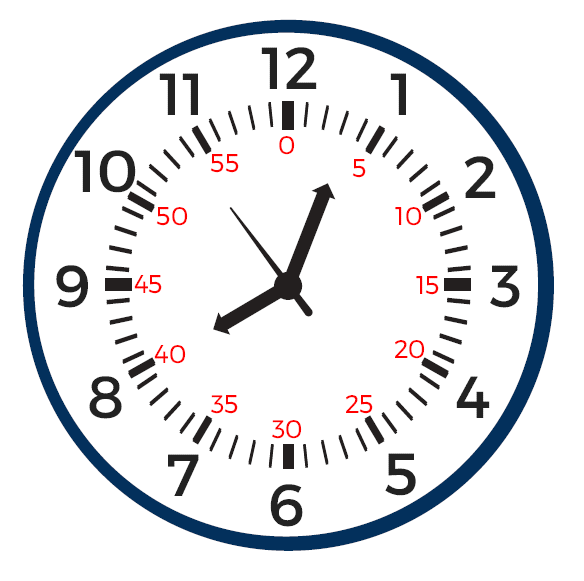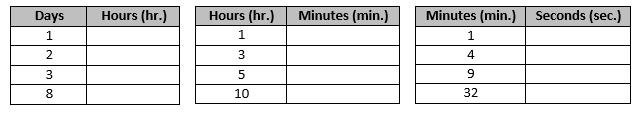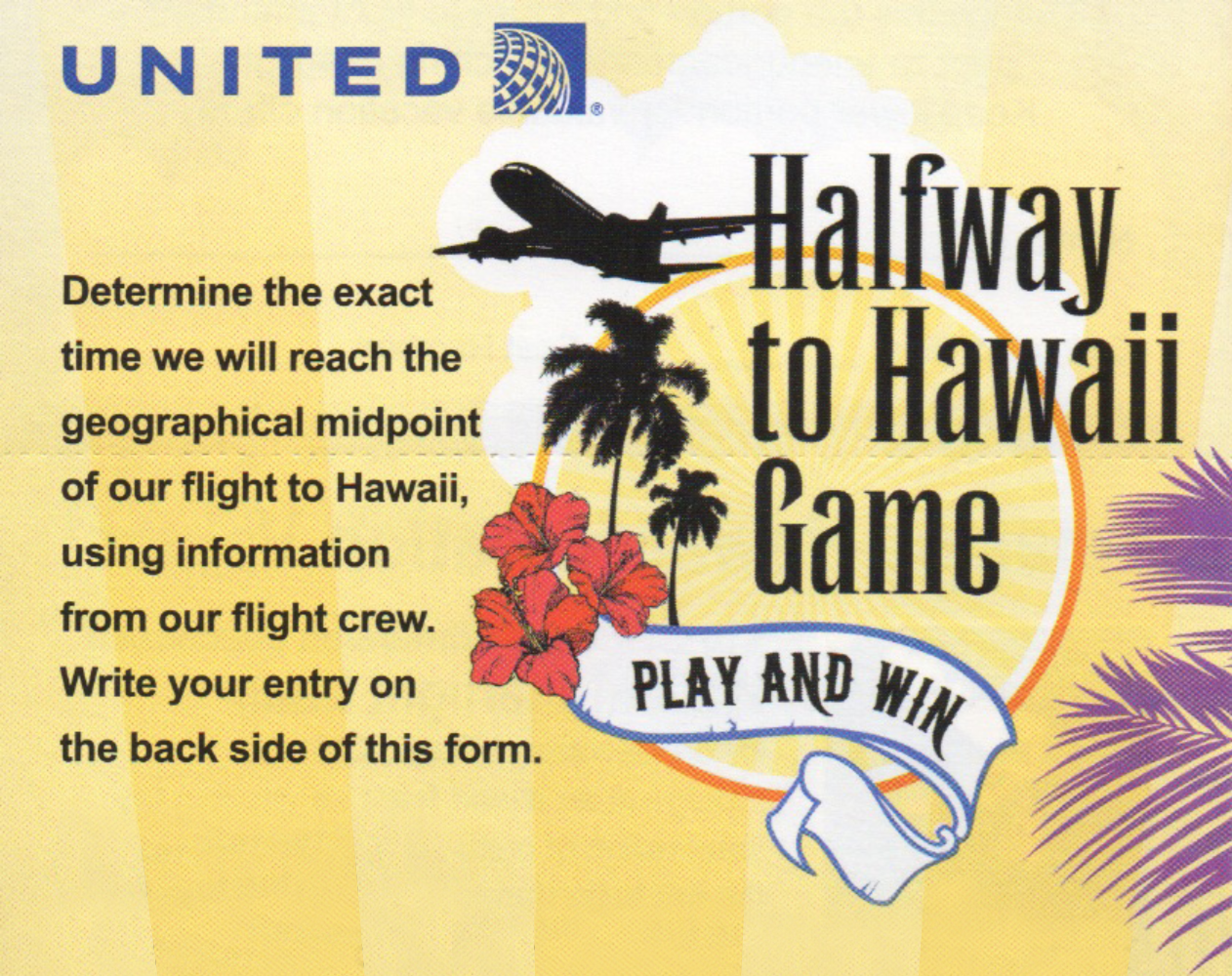# Unit Conversions

## Objective

Express time measurements in terms of a smaller unit, recording measurement equivalents in a two-column table. Solve one-step word problems that require time unit conversion.

## Common Core Standards

### Core Standards

?

• 4.MD.A.1 — Know relative sizes of measurement units within one system of units including km, m, cm; kg, g; lb, oz.; l, ml; hr, min, sec. Within a single system of measurement, express measurements in a larger unit in terms of a smaller unit. Record measurement equivalents in a two column table. For example, know that 1 ft is 12 times as long as 1 in. Express the length of a 4 ft snake as 48 in. Generate a conversion table for feet and inches listing the number pairs (1, 12), (2, 24), (3, 36), …

• 4.MD.A.2 — Use the four operations to solve word problems involving distances, intervals of time, liquid volumes, masses of objects, and money, including problems involving simple fractions or decimals, and problems that require expressing measurements given in a larger unit in terms of a smaller unit. Represent measurement quantities using diagrams such as number line diagrams that feature a measurement scale.

?

• 3.MD.A.1

• 4.OA.A.1

## Criteria for Success

?

1.  Establish benchmarks for the units of a day, an hour, a minute, and a second.
2. Understand that a day is 24 times longer than an hour, an hour is 60 times longer than a minute, and a minute is 60 times longer than a second.
3. Use these relationships to convert measurements from a larger time unit to a smaller unit (MP.7, MP.8).
4. Use these relationships to convert measurements from mixed time units to a smaller unit (MP.7, MP.8).
5. Solve one-step word problems that require time unit conversions, including problems that involve elapsed time (MP.4).

## Tips for Teachers

?

• The following material is needed for today's lesson: demonstration analog clock

Students have done extensive work with the relationships of time (2.MD.7a), how to read an analog clock (1.MD.3, 2.MD.7, 3.MD.1), and elapsed time (3.MD.1) in previous grades. Note, however, that 2.MD.7a is a Massachusetts-specific standard, but students are likely to know the relationships of time from real-world experience on their own.

#### Remote Learning Guidance

If you need to adapt or shorten this lesson for remote learning, we suggest prioritizing Anchor Task 2 (benefits from worked example)and Anchor Task 3 (can be done independently). You might decide not to give Anchor Task 4 independently, in which case it can be consolidated with Anchor Task 2 to be one Anchor Task. Find more guidance on adapting our math curriculum for remote learning here.

#### Fishtank Plus

• Problem Set
• Student Handout Editor
• Vocabulary Package

?

### Problem 1

a. How many seconds are in a minute?

b. How many minutes are in an hour?

c. How many hours are in a day?

d. How are each of these relationships represented on an analog clock? A picture of one is shown below.### Problem 2

Fill in the following converstion tables.### Problem 3

Anne, Matt, Cara, and Ross ran a half marathon but had staggered starts, so they aren’t sure who had the best time. They each recorded their run times in the following way:

 Person Time Anne 108 minutes 38 seconds Matt 2 hours 4 seconds Cara 6,873 seconds Ross 2 hours 4 minutes

### Problem 4

United Airlines has the following contest:You have the following flight information:

 Leave Wed, April 22 10:28 AM San Fransisco SFO 12:47 PM Honolulu HNL non-stop 5 hr 19 min total time

What would you submit as your contest entry?

#### References

101Questions Halfway to Hawaii

Halfway to Hawaii by Dan Meyer is made available on 101Questions under the CC BY 3.0 license. Accessed June 29, 2018, 11:47 a.m..

Modified by The Match Foundation, Inc.

## Problem Set & Homework

#### Discussion of Problem Set

• Look at #2(g) and #2(h). How many minutes are in a day? How many seconds are in a minute? How did you figure that out?
• What time did Juan arrive at his grandmother’s house in #8? How did you figure that out?
• How many minutes does Mr. Wilm stay at school in #10? How did you figure that out?
• Can anyone describe how time is kept in the military or in a foreign country?
• Is time (seconds, minutes, hours) defined as a metric or customary system?

?

### Problem 1

Solve.

a. 7 days = ____________ hours

b. 12 minutes 34 seconds = ____________ seconds

### Problem 2

Jacob needs to do his chores. It takes Jacob an hour and 45 minutes to mow the lawn and 20 minutes to clean his room. If he starts his chores at 2:00 PM, what time will he finish?

### Mastery Response

?